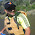# Add MathJax Beautiful Mathematic Notations for Blogger Blogs

Add beautiful & stunning math characters/ symbols/ notations for your blogs on Blogger made super easy by using MathJax javascript library.

Install MathJax javascript library/ plugin in just a few minutes & your math notations in your blog posts on Blogger will display so beautiful -- no reader setup required!

## What is MathJax?

Beautiful math in all browsers - A JavaScript display engine for mathematics that works in all browsers. No more setup for readers. It just works.

## MathJax Features & Benefits

High-quality typography

MathJax uses CSS with web fonts or SVG, instead of bitmap images or Flash, so equations scale with surrounding text at all zoom levels.

Modular Input & Output

MathJax is highly modular on input and output. Use MathML, TeX and ASCIImath as input and produce HTML+CSS, SVG and MathML as output.

Accessible & Usable

MathJax is compatible with screenreaders & provides zoom for everyone. You can also copy equations into Office, LaTeX, wikis, and other software.

## How to add MathJax for Blogs on Blogger?

• Go to Theme > Edit HTML
• Find <head> tag
• Paste below MathJax recommended setup underneath the <head> tag

<script type="text/x-mathjax-config">
//<![CDATA[
MathJax.Hub.Config({
tex2jax: {
inlineMath: [ ['$','$'], ["\$$","\$$"] ],
displayMath: [ ['$$','$$'], ["\$","\$"] ],
processEscapes: true
},
"HTML-CSS": { availableFonts: ["TeX"] }
});
//]]>
</script>
<script async="async" src="https://cdnjs.cloudflare.com/ajax/libs/mathjax/2.7.2/MathJax.js?config=TeX-AMS-MML_HTMLorMML" type="text/javascript">
</script>


• Click Save Theme

### Display Math Notations in Blog Posts

Now create a new posts to insert your mathematic characters. Using the above setup, any math characters you require to display using MathJax, enclose characters with the "\$" (without quotes) for inline display or " \$\$" (without quotes) for a block notation display. Below are examples:- This is a MathJax inline notation:$x^2$. More text after inline notations$x^2x^3$This is a MathJax inline notation:$x^2$. More text after inline notations$x^2x^3$ Below is a MathJax block notation: $$x^2$$ $$x^4$$ $$x^8$$ $$x^2$$ $$x^4$$ $$x^8$$  Quick Tips: Use backslash " \ " (without quotes) before a dollar sign to escape MathJax automated notations. Below are examples:- Escaped MathJax notations \$x^2\$\$x^2\$ Non escaped MathJax notations$x^2$$x^2\$

Hope you find this tutorial useful.

References:

1.Did not work for me. When I saved your code in my theme, it was changed. Frustratingly, when I tried to post it here, it was rendered correctly, so I took a screen shot which you can see here: https://drive.google.com/open?id=1DDrbQTrdnpoMLLut_ABCX3-6S8IuLt0t This has to do with representing special characters.

Also the code was rendered in black unlike all the other script, leading me to think it is not being recognized properly.

Any ideas?

1.Try to escape the javascript characters in Blogger Theme Edit HTML. Example as follows..

<script type="text/x-mathjax-config">
//<![CDATA[

//]]>
</script>

2.$$A=B^2$$

3.not work for me

1.It worked in the other Blogger blogs installed using the same concept above. Something might be missing perhaps... Here's the example Blogger site: Artie Gallery .

4.Thank you so much.

5.thank you so much

6.AnonymousJuly 17, 2020

Very nice

7.not working properly

8.Thanks for the tips. I've been playing around with it. How do you make those code blocks with a scroll bar?

I found a similar blog post but about using LaTeX. The script to edit the theme is a little different than what you use here. Can you please explain the differences between them?
9.